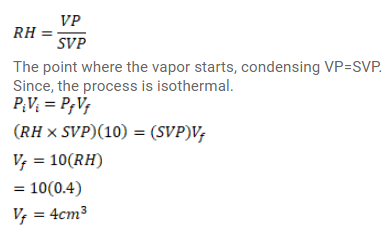# Use R=8.3 J/mol-K wherever required. Pure water vapor is trapped in a vessel ofQuestion:

Use $\mathrm{R}=8.3 \mathrm{~J} / \mathrm{mol}-\mathrm{K}$ wherever required.

Pure water vapor is trapped in a vessel of volume $10^{\mathrm{cm}^{3}}$. The relative humidity is $40 \%$. The vapour is compressed slowly and isothermally. Find the volume of vapour at which it will start condensing.

Solution: